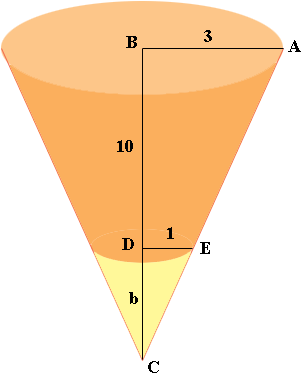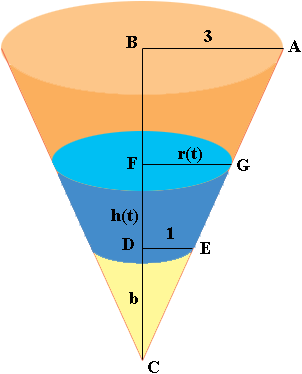SEARCH HOMEMath Central Quandaries & QueriesQuestion from Tim, a parent: A cup has a radius of 2" at the bottom and 6" on the top. It is 10" high. 4 Minutes ago, water started pouring at 10 cubic " per minute. How fast was the water level rising 4 minutes ago? How fast is the water level rising now? What will the rate be when the glass is full?Hi Tim,

I drew your cup (not to scale) and then extended it downwards to form a cone. The dimensions are in inches.Triangles $ABC$ and $EDC$ are similar and hence

$\frac{3}{10 + b} = \frac{1}{b}.$

Solving for $b$ gives $b = 5$ inches.

Now suppose that the water has been flowing into the cup for a while and the height of the water is $h(t)$ inches and the radius of the top of the water is $r(t)$ inches. $t$ is time in minutes.The volume of a cone of radius $r$ inches and height $h$ inches is $\frac13 \pi r^2 h$ cubic inches and hence, since $b = 5$ the volume of water in your cup $t$ minutes after the water begins to pour in is

$V(t) = \frac13 \pi \; r(t)^2 (h(t) + 5) - \frac13 \pi \;1^2 5.$

Can you complete the problem from here? Write back if you need more assistance.

PennyMath Central is supported by the University of Regina and The Pacific Institute for the Mathematical Sciences.